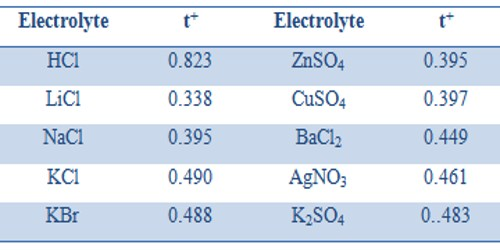Chemistry

# Which Factors are affecting Transference Number?The Transference number of ions varies with the change of concentration; such changes are quite complicated and the extent of change is also small. Transport number also depends on the temperature; t+ usually increases slightly with rising in temperature. Ascend in temperature is the key factor that affects a transport number. The anion transport number, therefore, generally decreases with the increase of temperature. With an increase in temperature, the transport number or transference number of cation and anion comes closer to 0.5. In the Table, the transport numbers of some cations are given.

Table: Cation Transport Number at 20°CDefinition: “The fraction of the total current carried by an ion is known as transport number, transference number or Hittorf number may be denoted by sets symbols like t+ and t or tc and ta or nc and na”.

For the same cation or the anion, the transport number depends on the electrolyte. Thus t+ values for K+ in KCl, KBr, and KNO3 are 0.490, 0.488 and 0.508 respectively. Similarly t values for Cl in HCl, LiCl, NaCl and KCl are 0.177, 0.662, 0.605 and 0.510 respectively. This is easy to understand because the transport number is not a unique property of the ion but depends on the relative speeds of the cation and anion in an electrolyte.

Unit of ionic mobility is Ohm-1 cm2 or V-1S-1cm2

Determination of transport number: Transport number can be determined by Hittorf’s method, moving boundary method, emf method, and ionic mobility.

Transport number and Ionic mobility: Ionic mobility or Ionic conductance is the conductivity of a solution containing 1 g ion, at infinite dilution, when two adequately huge electrodes are placed 1 cm apart.